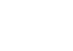write1. Q: What is this?

A: It’s a measuring cup.

2. Q: What do you use it for?

A: You use it for measuring things.

or

A: You use it for measuring liquids and dry ingredients.

3. What is this measuring cup made of?

4. Q: How many ounces are there in a cup?

A: There are eight ounces in a cup.

5. How many ounces are there in a half cup?

A: There are four ounces in a half cup.

6. Q: How many cups are there in a pint?

A: There are two cups in a pint.

7. Q: How many ounces are there in a pint?

A: There are 16 ounces in a pint.